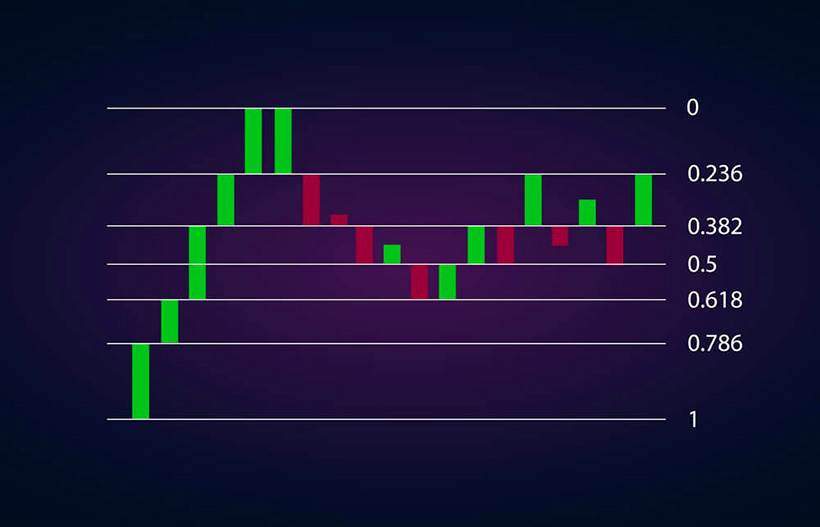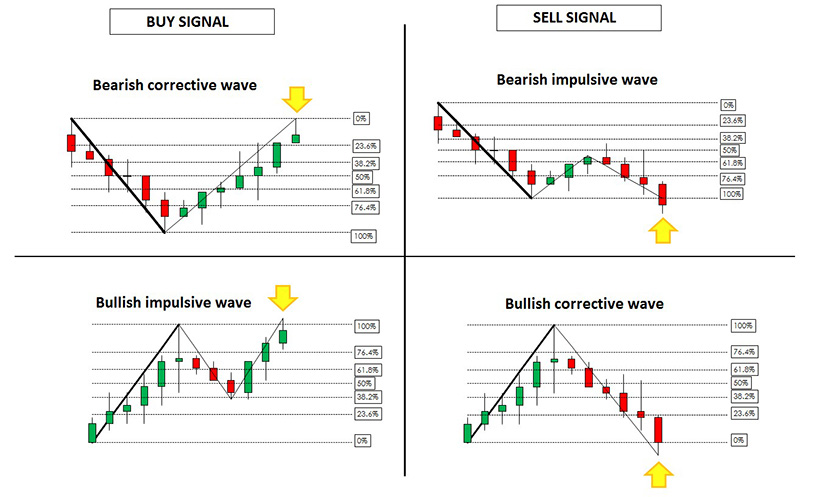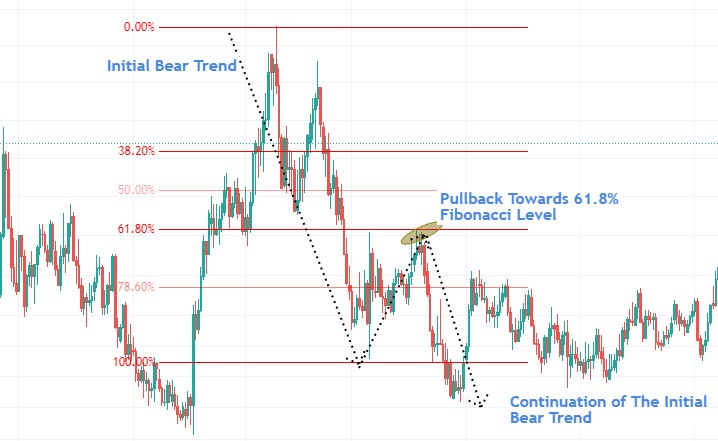Markets with a high level of volatility, such as foreign exchange, require special forecasting tools. The price that fluctuates in one range for a long time can suddenly fall or rise, and the struggle between bullish and bearish trends continues in a different price range. How to predict such a slide in the value of an asset and the dynamics of global markets in the short or long term? The ideal technique to perform this task is the Fibonacci sequence in Forex, which predicts the movement of the price chart, the levels of retracement, and expansion for a more profitable placement of orders. How does Fib retracement work in building Forex strategy?

## What Is Fibonacci Retracement? Simply Explained

Traders can apply Fibonacci in Forex differently, but more often, it is used to determine retracement levels. This allows you to find effective market entry points, set a stop loss, and perform other essential trading operations. How accurate is this technical analysis tool, and how to use Fibonacci retracement in Forex?### The Fibonacci Sequence

The sequence of Fibonacci numbers is actively used to model various processes both in the natural and social environment. This sequence is determined by stepwise summation of every two numbers, starting from zero, and can be calculated indefinitely. On its initial unfolding circle, it includes the following numbers: 0, 1, 1, 2, 3, 5, 8, 13, 21, 34, 55, 89, etc. Regarding trading, Forex Fibonacci is used to determine the fluctuation in the price of an asset. It is believed that economic and financial processes are also subject to the laws of nature, and therefore, the Forex Fibonacci strategy will be consistent with the natural rhythms of market expansion and contraction.### Fibonacci Retracement Levels

The ability to predict retracement levels in trading is just as important as being able to forecast trend reversals. After a steep rise or fall in the exchange rate, it is necessary for a trader to understand in what price range a temporary struggle for dominance between sellers and buyers will take place. Fibonacci levels in Forex set these fluctuation limits and indicate potential entry and exit points in the market. Therefore, the following scale is applied to the Fibonacci chart:

• 23.6%
• 38.2%
• 50%
• 61.8%
• 78.6%

At the same time, the 50% mark is not part of the Fibonacci Forex sequence, but it is plotted on the chart for other technical analysis operations and easier visual perception. This breakdown of the chart into levels is determined not manually but using the best Forex robots.How to use Fibonacci retracement in Forex? On the asset price chart, the maximum and minimum price values are selected and denoted as 100% and 0%. Price intervals that correspond to the golden ratio or the Fibonacci sequence make up the estimated support and resistance levels. They will be used in forecasting the price movement. Also, Fibonacci levels in Forex will help determine at what point traders may expect the end of a fluctuation or temporary retracement and should expect a trend reversal.

### Fibonacci Retracement indicators

How does Fibonacci retracement work to determine market entry points?

• When analyzing a bullish trend, Fibonacci indicators will show you three significant support levels, which will be at the points of 23.6%, 38.2%, and 61.8%.
• When analyzing a bear market, Fibonacci retracement Forex will indicate resistance levels on the same numerical values.

Therefore, if a trader wants to act not chaotically but in accordance with effective Forex strategies, they can wait until the price movement reaches Fibonacci forex levels and then use them to enter a position.## How to Use Fibonacci Retracement in Forex?

Fibonacci Forex trading strategy is considered so reliable that it is readily used by both beginners and professional traders. However, the latter ones do not use it recklessly but more carefully and take into account its weaknesses. Therefore, consider the heuristic value of this analytical tool but be aware of Fib Forex limitations.

What is Fibonacci retracement main value for which this technique is appreciated in all markets? Fibonacci retracement explained the laws of price fluctuations in the currency market, which made it possible to determine the levels to which the price returns. Among other undeniable advantages of using Fibonacci ratios, you will find the following:

• Suitable for analyzing both uptrends and downtrends;
• Correct visualization of estimated support and resistance levels;
• Can be used both with aggressive trading styles and passive waiting for price adjustments;
• Possibility to apply for predicting expansion levels, which set more distant prospects for price movement. In this case, the indicators 138.2% and 161.8% are used, which suggest the best time to enter or exit a position;
• Thanks to the addition of extension levels, you will be able to correctly place take-profit orders.• The Fibonacci retracement indicator relies only on price movement, and all other factors are simply ignored. In particular, such essential sources of price changes as news, reports, press releases, and other components of fundamental analysis are not taken into account.
• To get a stronger signal, Fibonacci forex trading must be combined with other indicators, such as candlestick analysis.

• Visualization;
• Predictive accuracy;
• Ease of use;
• Availability of a clear algorithm of actions;
• Universality in relation to market types;
• Possibility to use Forex robots in the Fibonacci trading system.